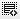Excel formulas1
Let me know the few more excel formulas?2

Syntax: =LEN(text)
Syntax: =PROPER(text)
Syntax: =TRIM(text)1

You can find more formulas infact all the formulas in excel itself.

From the menu bar select "FORMULAS" and then choose your formula which is categorised in various headings.0

You can find more formulas infact all the formulas in excel itself.

From the menu bar select "FORMULAS" and then choose your formula which is categorised in various headings.

Click on thisicon to add code snippet.